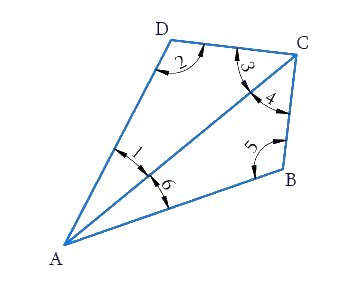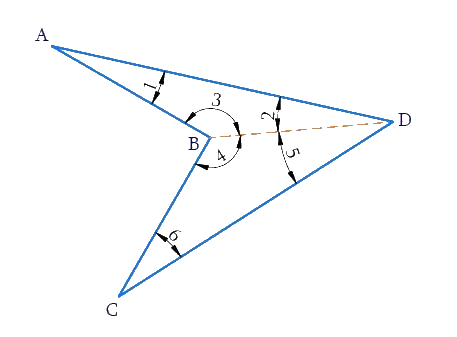In the verge of coronavirus pandemic, we are providing FREE access to our entire Online Curriculum to ensure Learning Doesn't STOP!

# Ex.3.1 Q3 Understanding Quadrilaterals Solution - NCERT Maths Class 8

Go back to  'Ex.3.1'

## Question

What is the sum of the measures of the angles of a convex quadrilateral? Will this property hold if the quadrilateral is not convex? (Make a non-convex quadrilateral and try!)

What is known?

Quadrilateral $$ABCD$$

What is unknown?

Sum of the measures of the angles of a convex quadrilateral.

Reasoning:

Let $$ABCD$$ be a convex quadrilateral. Then, we draw a diagonal $$AC$$ which divides the Quadrilateral into two triangles. We know that the sum of the angles of a triangle is $$180$$ degree, so by calculating the sum of the angles of a $$∆ABC$$ and , we can measure the sum of angles of convex quadrilateral.

Video Solution
Ex 3.1 | Question 3

## Text Solution$$ABCD$$ is a convex quadrilateral made of two triangles $$∆ABC$$ and $$∆ADC.$$ We know that the sum of the angles of a triangle is $$180$$ degree. So:

\begin{align}\!\angle 6\! +\! \angle 5 \!+ \!\angle 4\! =\! {180^ \circ }\left[ \begin{array}{l}{\rm{Sum}}\,\,{\rm{of}}\,{\rm{the}}\,{\rm{angles}}\,\\{\rm{of}}\,{\rm{\Delta ABC}}\,{\rm{ = 18}}{{\rm{0}}^{\rm{o}}}\end{array} \right]\\ \angle 1\!+ \!\angle2\!+\!\angle 3\!=\!{180^ \circ }\left[ \begin{array}{l}{\rm{Sum}}\,\,{\rm{of}}\,{\rm{the}}\,{\rm{angles}}\,\\{\rm{of}}\,{\rm{\Delta ADC}}\,{\rm{ = 18}}{{\rm{0}}^{\rm{o}}}\end{array} \right] \end{align}

\begin{align}\angle 6 &+ \angle 5 + \angle 4 + \angle 1 + \angle 2 + \angle 3\\ &= {{180}^{\rm{o}}} + {{180}^{\rm{\circ}}}\\ & = {{360}^{\rm{\circ}}}\end{align}

Hence, the sum of measures of the triangles of a convex quadrilateral is $$360^\circ$$. Yes, even if quadrilateral is not convex then, this property applies. Let $$ABCD$$ be a non-convex quadrilateral; join $$BD$$, which also divides the quadrilateral in two trianglesUsing the angle sum property of triangle, again$$ABCD$$ is a concave quadrilateral, made of two triangles $$\Delta \text{ABD}$$ and $$\Delta \text{BCD}$$ .Therefore, the sum of all the interior angles of this quadrilateral will also be,

$180^\circ + 180^\circ=360^\circ$

Learn from the best math teachers and top your exams

• Live one on one classroom and doubt clearing
• Practice worksheets in and after class for conceptual clarity
• Personalized curriculum to keep up with school